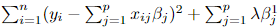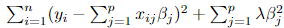# Lasso and Ridge Regression in Machine Learning

## Introduction

In this blog, we have discussed lasso and ridge, Regression models. The Lasso and Ridge linear regression models include a penalty (also called a regularization).  L1 regularisation is carried out by Lasso Regression, which adds a penalty equal to the absolute value of the magnitude of the coefficients. Ridge Regression performs L2 regularisation, which involves adding a penalty equal to the square of the coefficients’ magnitude.

## Lasso and Ridge Regression

### Lasso Regression

This regularisation method, also known as the penalized regression method, is used in feature selection using the shrinkage method. The Least Absolute Shrinkage and Selection Operator, or Lasso, is a regularisation and model selection technique. A model is referred to as lasso regression if the L1 regularisation method is used.

### Cost Function

The cost function of the Lasso regression is the Mean squared error + penalty factor. It can be represented by,where,

yi is a dependent variable

xi is independent variables

ß is co-efficient of regression

λ is the amount of shrinkage

The amount of shrinkage (or constraint) that will be applied to the equation is indicated by the penalty term λ. Lasso regression shrinks the coefficients and lowers multi-collinearity and model complexity. Any real-valued number between zero and infinity can be represented by λ  and the higher the value, the harsher the penalty.

When λ = 0, the coefficients will be the same as in simple linear regression.

when λ = ∞, the coefficients are going to be 0.  Anything less than zero will cause the objective to become infinite due to the infinite weighting of the square of coefficients.

### Ridge Regression

Ridge regression uses a penalty factor to impose a similar constraint on the coefficients as lasso regression does. However, ridge regression uses the square of the coefficients, whereas lasso regression uses their magnitude. L2 Regularization is just another name for ridge regression.

### Cost Function

The cost function of the Ridge regression is the Mean squared error + penalty factor. It can be represented by,where,

yi is a dependent variable

xi is independent variables

ß is co-efficient of regression

λ is the amount of shrinkage

Similar to Lasso regression, when λ = 0, the coefficients will be the same as in simple linear regression, and when λ = ∞, the coefficients are going to be 0.  Anything less than zero will cause the objective to become infinite due to the infinite weighting of the square of coefficients.

Limitations of Ridge and Lasso Regressions

Lasso regression will only keep a small number of variables and will set the other variables to zero when applied to a model with highly correlated variables. This will result in some information loss and decreased model accuracy.

The coefficients are reduced by ridge regression, but their values are never set to zero. The model will continue to be complex and retain all of its features, which could result in pretty poor model performance.

Also read: sklearn lasso and ridge models